ChemTalk

# What is Kw in Chemistry?## Core Concepts – Kw Equilibrium

In this article, you will learn about the concept of Kw in chemistry. Kw combines conceptual knowledge from branches like equilibrium, acids/bases, and properties of water to form a useful tool.

## Equilibrium and Disassociation

To understand the concept of Kw in chemistry, we need some background on equilibrium. In the world of chemistry, substances are constantly reacting with each other to form new substances. However, the reactions do not go on forever ; reactions either run out of reactants, or reach their equilibrium point. The equilibrium point is a ratio of the concentration of products to the concentration of reactants.

Liquids and solids don’t have true concentrations, so they aren’t included in these ratios. Each reaction has their own equilibrium point, and it can even change with temperature. For a more in-depth review of equilibrium, read ChemTalk’s article!

Another important topic to understand is disassociation. Some substances, when mixed with a solvent (usually water), will break apart into the ions they are made of. For example, when salt is dissolved in water it breaks apart into Na+ and Cl. We call this disassociating. If you want to learn more about which compounds disassociate the most, read ChemTalk’s article on strong acids and bases!

## What is Kw?

Kw is the equilibrium constant for the dissociation reaction of water’s ions. Kw is just like any other equilibrium constant, but instead of Keq , is written as Kw. This concept may sound confusing at first, but we can break it apart to make it simpler. The equilibrium constant is the ratio of product concentration to reactant concentration, while disassociation just references a molecule splitting apart in water.

Water dissociates like so: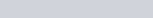Therefore, the equilibrium constant of water can be represented by the following equation: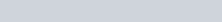Remember, H2O is not a factor in this equilibrium constant because it is a pure liquid. This means for every ten trillion water molecules, there is one pair of H+ and OH ions! When an acid or base is introduced to water, it can change the concentration of either of these ions, which in turn, changes the pH.

## Kw in pH Calculations

When calculating pH, we often reference the fact that the concentrations of H+ and OH always multiplies to 10-14. Water on its own is neutral, meaning the concentrations of H3O+ and OH are the same (10-7). The formula for the concentration of H+ ions can be written as such: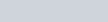If you need a refresher on how pH calculations work, give ChemTalk’s article on pH a read through! Sometimes, while doing practice problems, you’ll be given the concentration of OH ions instead of H+. Now that we have the conceptual knowledge of Kw, this isn’t a problem! We can simply divide the concentration of OH by the value of Kw (10-14) to get the concentration of H+.

## Practice Problem

Let’s do a quick practice problem to really nail the skill down.

An aqueous solution has a hydroxide ion concentration of 2.5 x 10⁻⁵ M. Calculate the pH of the solution. Is it acidic or basic?

To solve this problem, we first need to find the concentration of hydrogen ions in the solution. Since it is an aqueous solution, we can use Kw.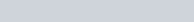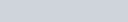Now, we can calculate the pH using the pH formula.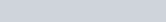Therefore, the pH of the solution is 9.4, and is basic.